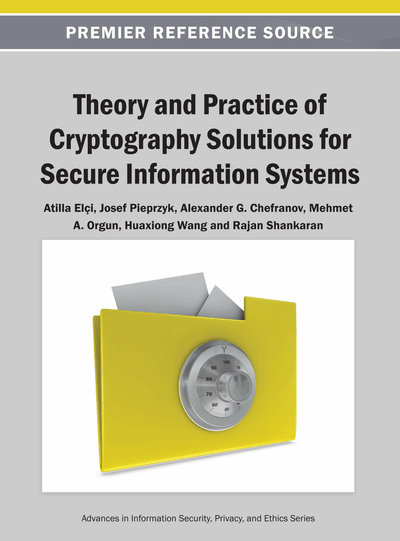# Encryption Schemes with Hyper-Complex Number Systems and Their Hardware-Oriented Implementation

Evgueni Doukhnitch (Istanbul Aydin University, Turkey), Alexander G. Chefranov (Eastern Mediterranean University, North Cyprus) and Ahmed Mahmoud (Al-Azhar University-Gaza, Palestine)
Copyright: © 2013 |Pages: 23
DOI: 10.4018/978-1-4666-4030-6.ch005
OnDemand PDF Download:
Available
\$37.50
No Current Special Offers

## Abstract

Quaternion Encryption Scheme (QES) is shown to be susceptible to the Known Plaintext-Ciphertext Attack (KPCA) due to improper choice of frame size and the procedure of secret quaternion updating. In this chapter, the authors propose a modification of the QES (M-QES) that is resistant to the KPCA. The M-QES is based on adjusting the frame size and the quaternion update procedure. An approach for effective hardware implementation of the proposed algorithm, HW-QES, is discussed. The HW-QES uses addition and shift operations. Extension of quaternion approach to another hyper-complex number systems, octonions, is used for designing a new hardware-oriented encryption algorithm, HW-OES. Experimental results show that the proposed M-QES and HW-QES are six-eight times more effective in the encryption quality of signals than the original QES. Additionally, M-QES and HW-OES are shown to be significantly more effective in the encryption quality of images than the original QES and well-known AES. The results show that the performance of the HW-QES is only 10% worse than that of QES.
Chapter Preview
Top

## Introduction

There are two hyper-complex number systems which are used in modern encryption systems: quaternions and octonions (Ward, 1997). The quaternion number system was discovered by a physicist Hamilton (1843) (Hamilton, 1847); it is an extension of the complex number system (so called hyper complex number). It has two parts, a scalar part and a vector part which is a vector in three-dimensional spaceSince the introduction of quaternion, it has been applied in several areas in computer science and engineering problems such as graphics and robotics (Kuipers 1999; Marins et al., 2001). It can be used to control rotations in three-dimensional space. The application of the quaternion number system is attractive in computation models due to its matrix representation. It has been applied as a mathematical model in encryption by several researchers. In (Nagase et al., 2004, 2005), a new Quaternion Encryption Scheme (QES) is proposed for signal encryption providing good hiding properties.

The octonion (Cayley numbers or octaves) number system was suggested by John T. Graves (1843) and discovered by Arthur Cayley (1845) (Ward, 1997). An octonion has two parts, a scalar part and a vector part which is a vector in seven-dimensional spaceIt is used in physics for 8-D rotation description and for quaternion valued matrix decomposition (Doukhnitch & Ozen, 2011). Recently in (Malekian & Zakerolhosseini, 2010), new encryption schemes based on non-associative octonion algebra were proposed for signal encryption with better security against lattice attack and/or more capability for protocol design.

Hyper-complex number based ciphers are attractive not only because they may be represented using matrix-vector multiplication but also that the inverse matrix for such transformation is a transpose of the original matrix. Matrix operations are rather simple and can be efficiently implemented that is especially important for multimedia data transmission.

(1) or, in the terms of plaintext-ciphertext matrices, (1) may be rewritten as (2) whereTransformation (2) may be also represented using matrix multiplication of the plaintext matrixby a secret key matrix depending onand producing the ciphertext matrixIt was expected that QES provides high security due to using dynamic key matrix obtained by changing the next quaternion components. But, this algorithm is a particular case of the well-known Hill cipher (HC) (Stallings, 2006). The HC is susceptible to the Known Plaintext-Ciphertext Attack (KPCA); therefore, QES can be broken with the KPCA, and the secret key matrix can be obtained.

## Complete Chapter List

Search this Book:
Reset